# Numerical Analysis of Slope Stability by Strength Reduction in Finite Elements Using ANSYS a Case Study of Qinglong-Xingyi Expressway Contract Section T1(K11+790~K11+875)

Numerical Analysis of Slope Stability by Strength Reduction in Finite Elements Using ANSYS a Case Study of Qinglong-Xingyi Expressway Contract Section T1(K11+790~K11+875)

Joan A. OnyangoChunyang Zhang

Jomo Kenyatta Unversity of Agriculture and Technology, P.O. BOX 62000-00200, Nairobi, Kenya

School of Resources and Environmental Engineering, Wuhan University of Technology, 205 Luoshi Road, Wuchang, Wuhan 430070, China

Corresponding Author Email:
jaonyango@jkuat.ac.ke
Page:
89-96
|
DOI:
https://doi.org/10.18280/eesrj.060206
13 March 2019
|
Accepted:
3 June 2019
|
Published:
30 June 2019
| Citation

OPEN ACCESS

Abstract:

This research aims at examining the stability condition of the slope on which Qinglong-Xingyi Expressway Contract Section T1(K11+790~K11+875) is built as well as put forward a suitable stabilization method. A 2D simulation model of the slope was developed for analysis using the Finite Element Software ANSYS. The main slip section (K11+850) was considered for developing the 2D model of the slope for simulation. The simulation results revealed a deep-seated failure surface in slip zone. The safety factor of the slope after the landslide was found to be 1.085, just around equilibrium. This meant that the slope was highly susceptible to failure in case of any trigger mechanisms hence reinforcement was inevitable in order to keep any eventuality at bay. Piles embedded in the bedrock were the best reinforcement method to contain the deep-seated failure surface developing in the sliding layer. Assurance of slope stability after reinforcement with piles is evident in the disappearance of the failure surface in the plastic strain plot, significant reduction in displacement, plastic strain and stress values. The findings of this research serve to strengthen numerical analysis by strength reduction as a tool for decision making in taking care of slope stability issues.

Keywords:

slope safety factor, landslide, slip zone, deep-seated failure, reinforcement, piles

1. Introduction

There is very little control by humans over the naturally occurring terrain that supports development infrastructure. Slopes have therefore to be carefully managed in order to ensure safety of humans and property. Slope management techniques have evolved over the years, and involves continuous monitoring to mitigate any eventuality that may result from slope instability. The stability analysis of natural and engineered slopes is a problem that is further complicated when there exists a slip zone whose shear strength behavior has to be taken into account . An in-depth knowledge of the stability condition of a slope is crucial for guaranteeing the safety of the humans as well property that are in close proximity to high slope faces . The phenomenal strength reduction of soil as the shear strength increases often leads to progressive slope failure characterized by a non-uniform mobilization of shear strength along the potential slip surface . A number of trigger factors such as inherent geological discontinuities, ground water conditions, strength of the rock mass, geotechnical parameters, dynamic forces, slope geometry, etc. contribute to ground failure by causing imbalance to natural forces .

The advancement of human engineering activities has seen increased complexities as railways, highways, water conservancies and mining facilities are built across slopes. Mine access roads as well as highways built by excavating into the relatively steep hillside and side casting the excavated materials to the outboard side are prone to slope instabilities . Slope distress is sometimes indicated by displacement of the guardrail posts, cracks and sinkholes on the hillside and road surface, small slumps occurring in the road cut on the inboard side, seeps developing near the toe of the embankment, and falling rock occurring from a nearby exposed rock face [6, 7]. These telltale signs, coupled with the effects of water seepage during rainy seasons, have caused landslides that in some cases have totally damaged the roads, some have had soils covering the roads rendering them impassable, the flowing material has damaged nearby houses and even lives lost . These have led to costly road closures and unscheduled repairs in a number of documented accidents. Rainfall being an important factor that induces instability in slopes, landslides often take place in hilly areas especially during rainy season .

That a slope may not necessarily be unstable after a landslide is a very well-known fact . There are a number of documented landslides such as the historical landslide in the Rječina River Valley, Croatia  which are dormant and have been stable for centuries because they haven’t felt any serious trigger factors. As pointed out in the CRC press , when material is moved the load on slope is reduced and some slopes become even more stable in this manner. Analyzing the stability condition of a slope after a landslide is crucial in assessing both short-term (often during construction) and long-term stability conditions of the slope, determining the slope sensitivity to different triggering mechanisms and also so as to do a redesign of failed slopes and where necessary, the planning and design of preventive and remedial measures.

Slope stability analysis makes use of soil and rock mechanics principles to investigate subsurface material conditions by determining the relevant physical, mechanical and chemical properties of these materials and their interaction with the engineering project at hand [14, 15]. The increasing demand for engineered cut and fill slopes on construction projects has only increased the need to understand analytical methods, investigative tools, and stabilization methods to solve slope stability problems . Growing experience on slope behavior over the decades has improved the understanding of the changes in soil properties that can occur over time. Improved analysis tools have also enabled recognition of the requirements and the limitations of laboratory and in situ testing for evaluating soil strengths as well as the principles of soil mechanics that relate soil behavior with slope stability . New and more effective instrumentation for monitoring slope behavior coupled with technologically advanced analytical procedures that enable extensive examination of the behavior of slope stability analyses have also been developed in the recent past . So far, the advancement of slope stability evaluation techniques has entered a more mature phase where experience and judgment, combined with improved understanding and more rational methods have improved the level of confidence in the results obtained through systematic observation, testing, and analysis .

Extensive research has gone into the analysis of the stability conditions of slopes with a number of quantitative studies being done for estimating the slope safety factor over the past few decades .

Potts et al [19, 20] contributed to the progressive failure analysis of slopes through their approach of simulating the strain-softening properties of brittle soils by reducing the strength parameters with the accumulated deviatoric plastic strains. Troncone  presented the results of a numerical study on a landslide in soils with strain-softening behavior that was triggered by deep excavations at the slope toe. He further extended the analysis into a three-dimensional problem  where the elastoviscoplastic model was adopted to account for the pronounced strain-softening behavior of the soil in the landslide. Other literature has also presented analyses of progressive failure based on elastic-plastic strain-softening constitutive models. Chai and Caeter  for instance employed the Modified Cam Clay Model to simulate the failure of an embarkment. Conte et al.  also used a similar approach to investigate slope failure cases triggered by weathering. A numerical method for evaluating the post failure large deformation of slopes was proposed by Samaneh and Taiebat . Besides the numerous numerical studies, numerous investigations have also been carried out on the residual strength of different types of slip zone soils by researchers like Skempton  and Chen and Liu . Mesri and Nejan’s  work in comparing residual strengths obtained in laboratory tests and those measured in the field has greatly improved the understanding of the mechanism of reactivation of ancient landslides.

Today, the traditional method of slope stability analysis mainly includes limit-equilibrium, limit analysis, slip-line etc. These methods based on the theory of limit-equilibrium cannot involve the stress-strain behavior of soil and need assumptions of failure surface shape (circular, log-spiral, piecewise linear, etc.) in advance . It typically restricted to Mohr-Coulomb soil models. The FEM method has many advantages over the traditional method in that;

•It not only satisfies the equilibrium condition of stress, but also involves the stress-strain relation.

•The critical failure surface is found automatically therefore no assumption needs to be made in advance about the shape or location of the failure surface.

•It can involve the non-linear elastic-plastic model, such as Mohr-Coulomb, Von Mises and Drucker-Prager model etc.

•It can monitor progressive failure up to and including overall shear failure.

•It can simulate the interaction between soil and support, such as pile and anchor etc.

•The result is more reliable.

The traditional FEM method just computes the stress field, displacement field and plastic zone. It cannot get the slope stability safety factor. With the development of computer techniques and the theory of generalized plastic mechanics of soil, the FEM Programs have made great progress in nonlinear finite element techniques . Its preprocessing and post-processing become more and more convenient.

Slope Safety Factor is a significant and reliable indicator of the stability condition of a slope. In order to overcome the challenges associated with traditional FEM methods of only computing the stress field, displacement field and plastic zone, this research makes use of FEM Program ANSYS which enables the computation of the slope safety factor by strength reduction. In this study, the stability analysis of Qinglong-Xingyi Expressway contract section T1(K11+790~K11+875) is done considering the strength reduction characteristics of the slip zone soil. The safety factor of the slope is equal to the reduction factor at which non-convergence occurs. A 2D model of the main slip section (K11+850) was selected for simulation with the aim of understanding the mechanical behavior of the slope and its response to reinforcement. The simulation results are compared with the actual reinforcement that was carried out on the slope. The authorities involved did a reinforcement to the slope using anti-slide piles of diameter 2 m and length of 23 m, at a spacing of 5m. Their decision was guided by measurements of residual sliding forces on the slope and well as the existence of a slip zone made up of highly weathered material. The findings of the numerical analysis results serve to strengthen numerical analysis by strength reduction as a tool to accurately guide on decision making regarding slope stability issues.

The remainder of this paper is organized as follows: Section 2 gives the geophysical properties of the area under study. Section 3 describes the general procedure for finite element analysis, the detailed steps used in this research as well as the simulation results. Section 4 outlines the conclusions and recommendations from this research.

2. Landslide Features

2.1 Location of the affected area

Qinglong-Xingyi Expressway is in Guizhou province, in the Qianxinan Buyei and Miao Autonomous Prefecture of China. Qinglong to Xingyi expressway is a component section of Bijie to Xingyi Expressway in six longitudinal of Guizhou province highway network planning, and the design mileage is 70.91 km. It lies between Latitude 24° N & Longitude 104° E towards Xingyi and Latitude 25° N and Longitude 105° E towards Qingong. Guizhou is a mountainous province lying at the eastern end of the Yungui Platau, although its higher altitudes are in the west and center. Guizhou has a subtropical humid climate, experiencing a few seasonal changes. Its annual average temperature is roughly 10 ℃ to 20 ℃, with January temperatures ranging from 1 ℃ to 10 ℃ and July temperatures ranging from 17 ℃ to 28 ℃. The annual average rainfall ranges between 26mm to 320mm, with the heaviest rain experienced in June. The Qing-Xing highway passes through Jianpo and Shuijing slopes. Junmin and Hui  studied the deformation characteristics of the entrance section of the mountain tunnel at Shajiaping in contract section T1, whose lithology is the same as the section T1(K11+790~K11+875) in this study.

2.2 Geology of the area

According to the engineering geological investigation report, the interior layer of the field area mainly consists of the following layers as indicated in Figure 1 and Figure 2.

Overburden layer: Variegated, composed of plastic containing gravel, silty clay and filling soil, depth ranging from 5.2~13.4 meters.

The whole strong weathering layer: It is 4.3~8.2 m thick, where the whole weathered layer has weathered into a soil shape, strong weathering layer, joint fracture development, rock fragmentation.

Bedrock: The Middle Permian Longtan Formation (P21) of argillaceous siltstone with carbonaceous mudstone and coal seam distribution in the landslide area.

The groundwater in the field area is mainly composed of quaternary unconsolidated fissure pore water, strong weathering fissure water and bedrock groundwater by precipitation recharge in rainy season, runoff to rainfall infiltration under furrow. Small part along the bedrock fissure to the low-lying excretion in weathered rock is aquifuge rock group, the geological survey found that appearing on both sides of the subgrade around. Small part along the weathered bedrock fissure to the low-lying excretion in weathered rock is aquifuge rock group, the geological survey found that appear on both sides of the embarkment, where S2, S3 is located in the former range of embarkment. Q=0.05~0.2 L/s. The location of the landslide leading edge and the shear exit is obvious, so the slip surface is clearer, the slip surface is steep, the gliding section is long, and the anti-sliding section is short, which leads to the residual sliding force. K11+850 was the main slip section considered in the geological study.

## 1.pngFigure 1. Schematic Longitudinal Geological Section K11+850

## 2.pngFigure 2. Schematic Transverse Geological Section

3. Stability Analysis

3.1 Finite element analysis

The numerical analysis of the slope stability was done using the finite element software ANSYS. In the ANSYS software, the Mohr-Coulomb (MC) failure surface is substituted by the Drucker-Prager (DP) failure cone, which is circumscribed to the MC hexagon pyramid. This is a pressure-dependent model for determining whether a material has failed or undergone plastic yielding. The criterion was introduced by Drucker and Prager  as an extended Von Mises Criterion. Both Drucker-Prager and Von Mises criteria were first developed for soils and considered the intermediate stress as well as the minimum and maximum principle stresses. The Drucker–Prager yield surface is basically a smooth version of the Mohr–Coulomb yield surface represented by Eq. (1) below:

$\mathrm{J}_{2}^{1 / 2}=\mathrm{k}+\alpha \mathrm{J}_{1}$         (1)

$\mathrm{J}_{1}$ and $\mathrm{J}_{2}$ are stress invariants expressed as Eq. (2) and Eq. (3) below:

$J_{1}=\sigma_{1}+\sigma_{2}+\sigma_{3}$        (2)

$J_{2}=\frac{1}{6}\left[\left(\sigma_{1}-\sigma_{2}\right)^{2}+\left(\sigma_{1}-\sigma_{3}\right)^{2}+\left(\sigma_{2}-\sigma_{3}\right)^{2}\right]$     (3)

k and α express cohesion and internal friction properties of the intact rock respectively. By setting α=0, this criterion reduces to Von Mises Criterion. The Drucker-Prager criterion has two forms: The Inscribed Drucker-Prager and Circumscribed Drucker-Prager criterion. Colmenares and Zoback  outlined some equations that express the relationship between cohesion, internal friction, and k & α for the two types of Drucker-Prager criteria. The Inscribed Drucker-Prager criterion is represented by Eq. (4) and Eq. (6) while Circumscribed Drucker-Prager criterion is represented by Eq. (5) and Eq. (7).

$\alpha_{\mathrm{Ins}}=\frac{3 \sin \varphi}{\sqrt{9+3 \sin ^{2} \varphi}}$        (4)

$\alpha_{\mathrm{Cir}}=\frac{6 \sin \varphi}{\sqrt{3}(3-\sin \varphi)}$         (5)

$\mathrm{k}_{\mathrm{Ins}}=\frac{3 \sigma_{\mathrm{c}} \cos \varphi}{2 \tan \left(45+\frac{\varphi}{2}\right) \sqrt{9+3 \sin ^{2} \varphi}}$        (6)

$\mathrm{k}_{\mathrm{Cir}}=\frac{\sqrt{3} \sigma_{\mathrm{c}} \cos \varphi}{\tan \left(45+\frac{\varphi}{2}\right) \sqrt{3-\sin \varphi}}$          (7)

From the point of view of numerical efficiency, this brings much convenience to numerical calculation.

3.2 Finite element model

The slope model, Figure 3, is treated as an elastic-perfectly plastic model (Drucker-Prager Model). This model requires the following five input parameters which need to be defined for each of the different material layers making up the slope: density γ, young's modulus E, Poisson's ratio ν, cohesion c and friction angle φ.

The PLANE82 element is chosen for representing the slope material. The 8-node element is defined by eight nodes having two degrees of freedom at each node i.e. translations in the nodal x and y directions. The element may be used as a plane element or as an axisymmetric element. The element has plasticity, creep, swelling, stress stiffening, large deflection, and large strain capabilities. These properties make the element quite a suitable choice for representing the soil and rock mechanical properties.

## 3.png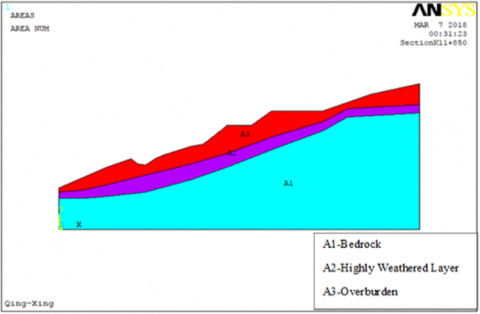Figure 3. Slope model

A 2D-model of the slope was developed from the combination of the AutoCAD drawings of the topography and the sectional drawings in Figure 1and Figure 2 using ANSYS to represent the three major material layers forming the strata. The physico-mechanical properties of the materials used in this analysis are in Table 1.

Table 1. Physico-mechanical input parameters

 Rock Mass Over-burden Strongly weathered layer Bed rock Dry Unit Weight(kg/m3) 1900 2000 2200 Modulus of Elasticity, E(Mpa) 200 300 4500 Poisson’s Ratio 0.3 0.29 0.26 Cohesion, C(KPa) 34 13.8 117.6 Friction Angle, Φ (0) 17 11.5 23

3.3 Analysis procedure

The 2D model was meshed as in Figure 4 with the sliding layer being represented with finer mesh so as to increase the accuracy of the results for the stresses and strains that develop. The model was constrained in the X-direction at the sides and both X&Y directions at the bottom. Gravity load was applied to simulate the self-weight of the slope. The applied load is the self-weight of the slope which is applied as gravity in the positive Y direction. The standard earth gravity can only be defined along one of the three global coordinate system axes and since the standard earth gravity is defined as an acceleration, its direction is defined opposite to that of gravitational force. The ACEL command in ANSYS applies an acceleration field (not gravity) to a body. Therefore, to apply gravity to act in the negative Y direction, a positive Y acceleration is specified. Slope stability analysis is carried out as a non-linear static structural analysis. A surcharge load of 20kN/m2 was applied in the analysis.

In this study, the slope stability was determined by strength reduction method (SRM), a principle which was proposed by Matsui and San  and has been widely applied by a number of researchers in their analyses. To calculate the factor of safety of the slope using the SRM, a strength reduction factor (SRF) is first chosen for the reduction of material strength properties cohesion and friction angle (c-φ reduction). Simulations are then run for a series of the symmetrically reduced shear strength parameters which are calculated as given in Eq (8) and eq (9) below:

$c_{r}=\frac{1}{S R F} c$       (8)

$\varphi_{\mathrm{r}=\arctan \left(\frac{1}{\mathrm{SRF}} \tan \varphi\right)}$               (9)

During the numerical analysis, $c_{r}$ and $\varphi_{r}$ are used as the basic input parameters. The trial factor of safety SRF is incremented until the convergence criterion is not satisfied. In this case, the slope is in the limit equilibrium state, and the corresponding SRF is considered as the real factor of safety (FOS).

Slope stability/instability is determined by the convergence or nonconvergence of the solution, the displacement of the slope (a sharp increase in displacement means instability) and development of a plastic zone.

## 4.png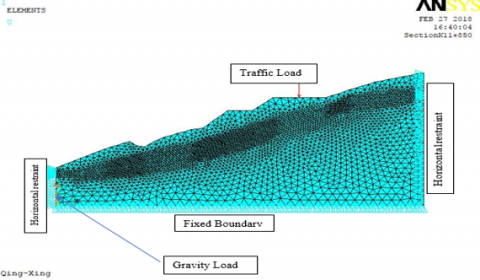Figure 4. Finite element mesh and boundary conditions used in the numerical analysis

3.4 Numerical analysis results

3.4.1 Stability condition of slope before reinforcement

## 5.png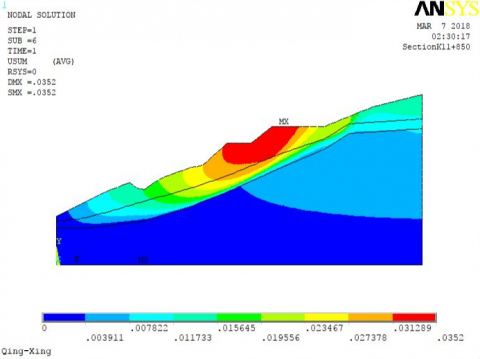Figure 5. Displacement Vector Sum with gravity load only

In the initial state, before strength reduction, the slope undergoes some deformation under the effect of self-gravity as well as traffic load. Under the effect of self-gravity alone, the maximum displacement is 0.0352 m (Figure 5) while a combination of self-gravity and traffic load yields a displacement of 0.040025 m (Figure 6). The Von Mises plastic strain for self-gravity was 0.014465 (Figure 7) and that for a combination of self-gravity and traffic load was 0.020677 (Figure 8). This shows that traffic load has an effect on the stability of the slope. Surcharge (traffic) load of 20kN/m2 is therefore considered in the analysis.

## 6.pngFigure 6. Displacement Vector Sum with gravity & surcharge load

## 7.pngFigure 7. Plastic Strain with gravity load only

## 8.pngFigure 8. Plastic Strain with gravity and surcharge load

The analysis of the dry slope yielded a safety factor (SF) of 1.085, which is equal to the reduction factor at which failure occurs. This SF value is way below the minimum allowable factor of safety against sliding of 1.5 that is recommended for the stability of cut slopes in fine-grained soils. The plot of the first principal stress gives a maximum positive stress value of 59.285kPa (Figure 9) occurring at the topmost part of the slope. This means the slope is experiencing tensile stresses at the top. At the point of failure, the maximum displacement expected is 0.06797m(Figure 10) experienced around the road section. The displacement vector plot (Figure 11) shows a downward motion of the overburden up to the sliding layer beneath. This therefore means that the support mechanism must take care of the sliding. The Von Mises plastic strain plot (Figure 12) shows a deep-seated failure surface developing within the highly weathered sliding layer. The low safety factor value leads to a conclusion that the slope is highly susceptible to failure in case of trigger mechanisms and hence a means for reinforcement must be put in place.

## 9.png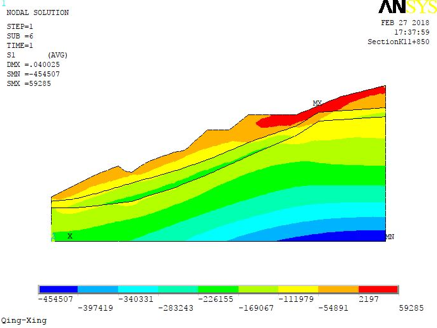Figure 9. First Principal Stress at SRF=1.085

3.4.2 Slope stabilization

From the analysis results, it’s clear that the slope stability is just around equilibrium hence highly likely to fail in case of any trigger factors. The presence of a sliding layer means that the reinforcement mechanism must be anchored within the bedrock so as to contain the sliding. In this study, prestressed steel reinforced concrete spun piles of 1m diameter and length 22m were chosen for reinforcement in order to increase the resistance to sliding.

## 10.png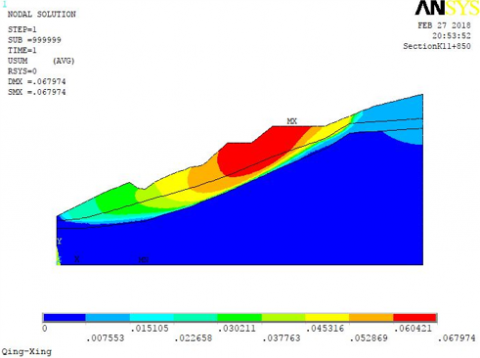Figure 10. Displacement Vector Sum at SRF=1.085

## 11.pngFigure 11. Displacement Vector at SRF=1.085

## 12.pngFigure 12. Von Mises Plastic Strain at SRF=1.085

With a single row of piles in place, the maximum displacement value reduced significantly to 0.001554 m (Figure 13), the maximum plastic strain also reduced to 4.53e-4 (Figure 14). The strain within the sliding section is completely eliminated. The first principal stress plot (Figure 15) shows the top strata of the slope are under compressive stress after the pile is installed. The maximum stress plotted is however high, 255.617 kPa, developing at the bottom of the pile because when the pile is in place, the pressure bulb extends to a considerable depth below the base of the pile. The displacement vector plot (Figure 16) shows there’s very low likelihood of downward movement of the slope.

## 13.pngFigure 13. Displacement Vector Sum

## 14.pngFigure 14. Von Mises plastic strain

## 15.pngFigure 15. First principal stress

## 16.png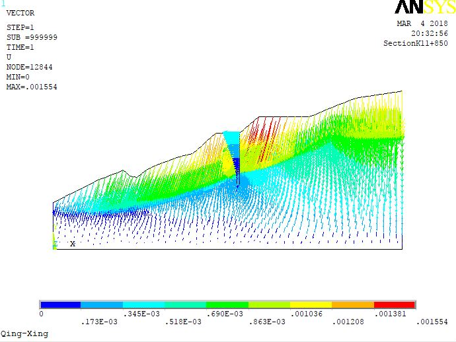Figure 16. Displacement vector plot

4. Conclusions and Recommendations

The application of finite element analysis using ANSYS has seen the determination of the safety factor of the Qinglong-Xingyi Expressway slope and its stability condition after the rainfall induced landslide. Based on the analysis, the following conclusions and recommendations are drawn:

a) The slope safety factor of 1.085 indicate a slope that is highly susceptible to failure. Reinforcement with a single row of piles gives an assurance of a stable slope. This is evident from the absence of the failure surface in the plot of the plastic strain, as well as significant reduction in the values of displacement, plastic strains and stresses. Slope reinforcement with piles is suitable especially due to the existence of a deep-seated slip zone.

b) The simulation results are comparable with the actual reinforcement measure that was done on the slope. Numerical analysis by strength reduction can therefore be confidently relied upon to aid in decision making on slope stability issues.

The safety factor value is a significant indicator of the stability condition of a slope. It was however not achieved for the reinforced slope due to simulation challenges that caused the solution to halt. Further work is required on the selection of the most suitable elements for representation of the interaction between piles and rock mass as well as applicable boundary conditions to ensure the convergence of the solution. This in effect will further build confidence in the numerical analysis results.

Nomenclature
 c cohesion, Nm-2 J deviatoric stress invariant k material constant expressing internal friction properties of the intact rock, varies with the value of α SRF Strength reduction factor Greek symbols $\alpha$ material constant expressing internal friction properties of the intact rock $\sigma$ principal stress, Nm-2 $\varphi$ angle of friction, degrees Subscripts Ins Inscribed Cir Circumscribed
References

 Chen, X.P., Zhu, H.H., Huang, J.W., Liu, D. (2016). Stability analysis of an ancient landslide considering shear strength reduction behavior of slip zone soil. Landslides, 13(1): 173-181. http://dx.doi.org/10.1007/s10346-015-0629-7

 Abramson, L.W., Lee, T.S., Sharma, S., Boyce, G.M. (2002). Slope Stability and Stabilization Methods. John Wiley & Sons, New York, USA.

 Nagaraj, T., Srinivasa, B. (1994). Analysis and Prediction of soil Behaviour. Taylor & Francis, New Delhi, India.

 Froude, M. (2011). Capturing and characterising pre-failure strain on failing slopes. Masters Thesis. Durham University, UK.

 Donnelly, L.B. (2006). The mam tor landslide, geology & mining legacy around Castleton, Peak District National Park, Derbyshire, UK. In Engineering Geology for Tomorrow's Cities, Proceedings of the 10th Congress of the International Association for Engineering Geology and the Environment, Nottingham, UK.

 Plaxico, C., Patzner, G., Ray, M. (1998). Finite-element modeling of guardrail timber posts and the post-soil interaction. Transportation Research Record: Journal of the Transportation Research Board, 139-146. http://dx.doi.org/10.3141/1647-17

 Choubey, V., Bartarya, S., Ramola, R. (2005). Radon variations in an active landslide zone along the Pindar River, in Chamoli District, Garhwal Lesser Himalaya, India. Environmental Geology, 47(6): 745-750. http://dx.doi.org/10.1007/s00254-004-1196-8

 Assefa, E., Lin, L.J., Sachpazis, C.I., Feng, D.H., Shu, S.X., Anastasiadis, A. (2016). Discussion on the analysis, prevention and mitigation measures of slope instability problems: A case of Ethiopian railways. Electronic Journal of Geotechnical Engineering, 21(12): 4101-4119.

 Brand, E.W., Premchitt, J., Phillipson, H. (1984). Relationship between rainfall and landslides in Hong Kong. In Proceedings of the 4th International Symposium on Landslides. Toronto, Canada.

 Guzzetti, F., Carrara, A., Cardinali, M., Reichenbach, P. (1999). Landslide hazard evaluation: A review of current techniques and their application in a multi-scale study, Central Italy. Geomorphology, 31(1): 181-216. http://dx.doi.org/10.1016/S0169-555X(99)00078-1

 Dugonjić Jovančević, S., Peranić, J., Ružić, I., Arbanas, Ž. (2016). Analysis of a historical landslide in the Rječina River Valley, Croatia. Geoenvironmental Disasters, 3(1): 26. http://dx.doi.org/10.1186/s40677-016-0061-x

 Hoek, E., Bray, J.D. (2014). Rock slope engineering. CRC Press. http://dx.doi.org/10.1201/9781482267099

 Corominas, J., van Westen, C., Frattini, P., Cascini, L., Malet, JP., Fotopoulou, S., Catani, F., Van Den Eeckhaut, M., Mavrouli, O., Agliardi, F. (2014). Recommendations for the quantitative analysis of landslide risk. Bulletin of Engineering Geology and the Environment, 73(2): 209-263. http://dx.doi.org/10.1007/s10064-013-0538-8

 Terzaghi, K., Peck, R.B., Mesri, G. (1996). Soil mechanics in engineering practice. John Wiley & Sons. http://hdl.handle.net/123456789/123145

 Lancellotta, R. (2008). Geotechnical Engineering. CRC Press. https://doi.org/10.1201/9781482265934

 Fredlund, D., Krahn, J. (1977). Comparison of slope stability methods of analysis. Canadian Geotechnical Journal, 14(3): 429-439. http://dx.doi.org/10.1139/t77-045

 Eberhardt, E. (2003). Rock slope stability analysis-Utilization of advanced numerical techniques. Earth and Ocean Sciences at UBC Report. University of British Columbia (UBC), Vancouver, Canada, pp. 41.

 Dreyfus, H., Dreyfus, S.E., Athanasiou, T. (2000). Mind over machine. Simon and Schuster. https://ieeexplore.ieee.org/stamp. Accessed on Jan 10, 2019.

 Potts, D., Dounias, G., Vaughan, P. (1990). Finite element analysis of progressive failure of Carsington embankment. Géotechnique, 40(1): 79-101. https://doi.org/10.1680/geot.1990.40.1.79

 Potts, D., Kovacevic, N., Vaughan, P. (2009). Delayed collapse of cut slopes in stiff clay, in Selected papers on geotechnical engineering by PR Vaughan. Thomas Telford Publishing, pp. 362-391. http://dx.doi.org/10.1680/spogebprv.36208.0018

 Troncone, A. (2005). Numerical analysis of a landslide in soils with strain-softening behaviour. Geotechnique, 55(8): 585-596. http://dx.doi.org/10.1680/geot.2005.55.8.585

 Troncone, A., Conte, E., Donato, A. (2014). Two and three-dimensional numerical analysis of the progressive failure that occurred in an excavation-induced landslide. Engineering Geology, 183: 265-275. http://dx.doi.org/10.1016/j.enggeo.2014.08.027

 Chai, J., Carter, J.P. (2009). Simulation of the progressive failure of an embankment on soft soil. Computers and Geotechnics, 36(6): 1024-1038. http://dx.doi.org/10.1016/j.compgeo.2009.03.010

 Conte, E., Silvestri, F., Troncone, A. (2010). Stability analysis of slopes in soils with strain-softening behaviour. Computers and Geotechnics, 37(5): 710-722. http://dx.doi.org/10.1016/j.compgeo.2010.04.010

 Mohammadi, S., Taiebat, H.A. (2013). A large deformation analysis for the assessment of failure induced deformations of slopes in strain softening materials. Computers and Geotechnics, 49: 279-288. http://dx.doi.org/10.1016/j.compgeo.2012.08.006

 Skempton, A.W. (1985). Residual strength of clays in landslides, folded strata and the laboratory. Geotechnique, 35(1): 3-18. http://dx.doi.org/10.1680/geot.1985.35.1.3

 Chen, X., Liu, D. (2014). Residual strength of slip zone soils. Landslides, 11(2): 305-314. http://dx.doi.org/10.1007/s10346-013-0451-z

 Mesri, G., Huvaj-Sarihan, N. (2012). Residual shear strength measured by laboratory tests and mobilized in landslides. Journal of Geotechnical and Geoenvironmental Engineering, 138(5): 585-593. https://doi.org/10.1061/(ASCE)GT.1943-5606.0000624

 Yingren, Z.S.Z. (1999). Slope safety factor analysis using ANSYS. Logistical Engineering University. Chong Qing, China. https://scholar.google.com/scholar. Accessed Jan 27, 2019.

 Noor, A.K. (1981). Survey of computer programs for solution of nonlinear structural and solid mechanics problems. In Computational Methods in Nonlinear Structural and Solid Mechanics, Elsevier, pp. 425-465. https://doi.org/10.1016/B978-0-08-027299-3.50051-0

 Junmin, H., Hui, D. (2014). Characteristics analysis about surrounding rock deformation of entrance section in mountain tunnel. Chinese Journal of Underground Space and Engineering, 10(4): 818-822. https://wenku.baidu.com/view/b8b915632cc58bd63086bd73.html. Accessed Feb 12, 2019.

 Drucker, D.C., Prager, W. (1952). Soil mechanics and plastic analysis or limit design. Quarterly of Applied Mathematics, 10(2): 157-165. http://dx.doi.org/10.1090/qam/48291

 Colmenares, L., Zoback, M. (2002). A statistical evaluation of intact rock failure criteria constrained by polyaxial test data for five different rocks. International Journal of Rock Mechanics and Mining Sciences, 39(6): 695-729. http://dx.doi.org/10.1016/S1365-1609(02)00048-5

 Matsui, T., San, K.C. (1992). Finite element slope stability analysis by shear strength reduction technique. Soils and Foundations, 32(1): 59-70. http://dx.doi.org/10.3208/sandf1972.32.59